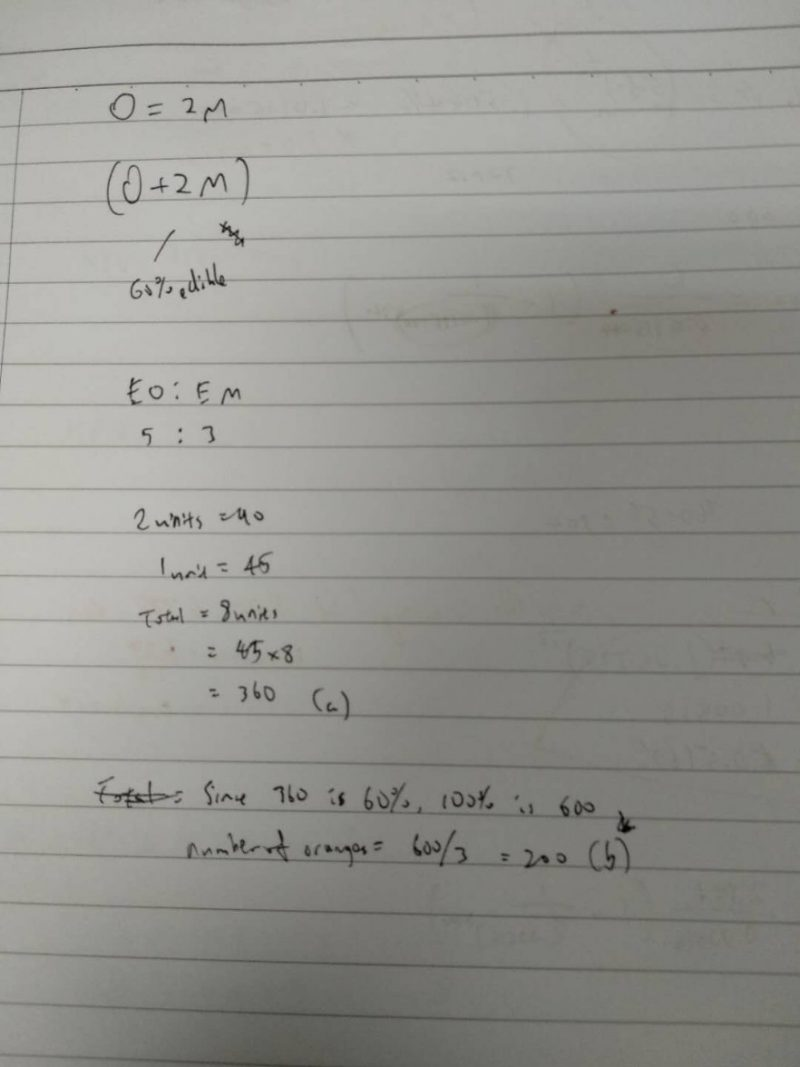# Question

Mr Tham picked twice as many oranges as mangoes from his farm. While sorting out the fruits, he found that only 60% of the fruits are edible. The ratio of the number of edible oranges to the number of edible mangoes was 5:3. There were 90 more edible oranges than edible mangoes.

(a) What was the total number of edible oranges and mangoes?

(b) How many oranges did Mr Than pick at first?

Source: Assesment

(a)
edible oranges : edible mangoes
5 : 3

5 – 3 = 2
2 units = 90
1 unit = 45
5 + 3 = 8
8 units = 8 x 45 = 360

(b)
60% ——-> 360
1% ——-> 360/60 = 6
100% ——-> 100 x 6 = 600
600 x 2/3 = 400

Ans : (a) 360 edible oranges and mangoes; (b) 400 oranges.

0 Replies 0 Likes0 Replies 1 Like